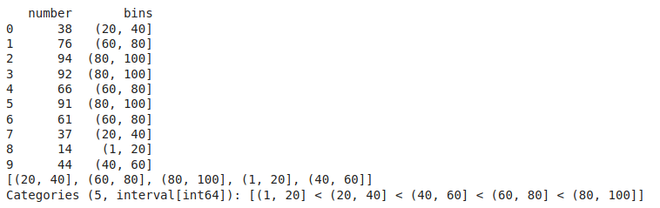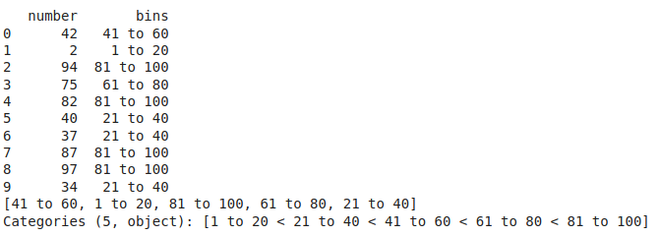# Pandas.cut() method in Python

• Difficulty Level : Basic
• Last Updated : 13 Jul, 2021

Pandas cut() function is used to separate the array elements into different bins . The cut function is mainly used to perform statistical analysis on scalar data.

Syntax: cut(x, bins, right=True, labels=None, retbins=False, precision=3, include_lowest=False, duplicates=”raise”,)

Parameters:

x: The input array to be binned. Must be 1-dimensional.

bins: defines the bin edges for the segmentation.

right : (bool, default True )  Indicates whether bins includes the rightmost edge or not. If right == True (the default), then the bins [1, 2, 3, 4] indicate (1,2], (2,3], (3,4].

labels : (array or bool, optional)  Specifies the labels for the returned bins. Must be the same length as the resulting bins. If False, returns only integer indicators of the bins.

retbins : (bool, default False) Whether to return the bins or not. Useful when bins is provided as a scalar.

Example 1: Let’s say we have an array of 10 random numbers from 1 to 100 and we wish to separate data into 5 bins of (1,20] , (20,40] , (40,60] ,  (60,80] , (80,100] .

## Python3

 `import` `pandas as pd``import` `numpy as np`  `df``=` `pd.DataFrame({``'number'``: np.random.randint(``1``, ``100``, ``10``)})``df[``'bins'``] ``=` `pd.cut(x``=``df[``'number'``], bins``=``[``1``, ``20``, ``40``, ``60``,``                                          ``80``, ``100``])``print``(df)` `# We can check the frequency of each bin``print``(df[``'bins'``].unique())`

Output:Example 2: We can also add labels to our bins, for example let’s look at the previous example and  add some labels to it

## Python3

 `import` `pandas as pd``import` `numpy as np` `df ``=` `pd.DataFrame({``'number'``: np.random.randint(``1``, ``100``, ``10``)})``df[``'bins'``] ``=` `pd.cut(x``=``df[``'number'``], bins``=``[``1``, ``20``, ``40``, ``60``, ``80``, ``100``],``                    ``labels``=``[``'1 to 20'``, ``'21 to 40'``, ``'41 to 60'``,``                            ``'61 to 80'``, ``'81 to 100'``])` `print``(df)` `# We can check the frequency of each bin``print``(df[``'bins'``].unique())`

Output:My Personal Notes arrow_drop_up